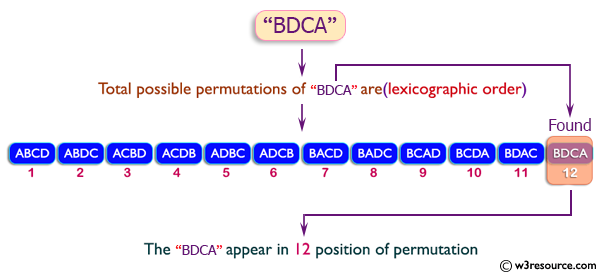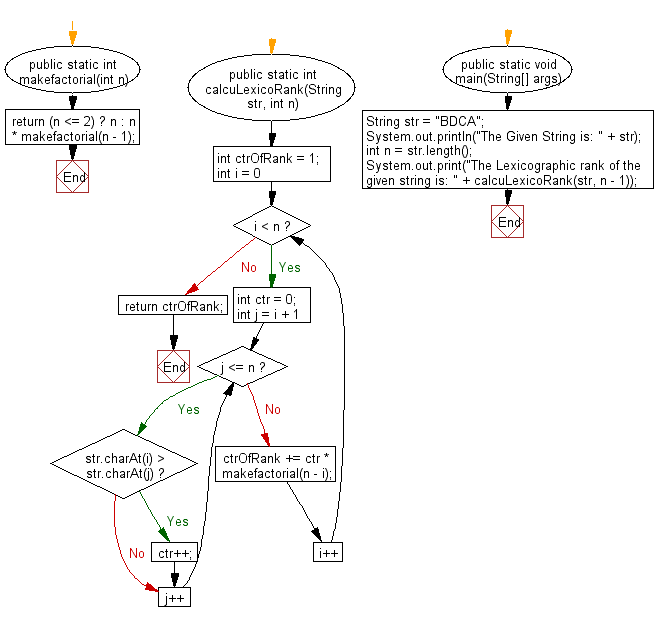﻿ Java exercises: Find lexicographic rank of a given string - w3resource# Java String Exercises: Find lexicographic rank of a given string

## Java String: Exercise-50 with Solution

Write a Java program to find lexicographic rank of a given string.

Pictorial Presentation:Sample Solution:

Java Code:

``````import java.util.*;
class Main {
public static int makefactorial(int n) {
return (n <= 2) ? n : n * makefactorial(n - 1);
}
public static int calcuLexicoRank(String str, int n) {
int ctrOfRank = 1;
for (int i = 0; i < n; i++) {
int ctr = 0;
for (int j = i + 1; j <= n; j++) {
if (str.charAt(i) > str.charAt(j))
ctr++;
}
ctrOfRank += ctr * makefactorial(n - i);
}
return ctrOfRank;
}
public static void main(String[] args) {
String str = "BDCA";
System.out.println("The Given String is: " + str);
int n = str.length();
System.out.print("The Lexicographic rank of the given string is: " + calcuLexicoRank(str, n - 1));
}
}
```
```

Sample Output:

```The Given String is: BDCA
The Lexicographic rank of the given string is: 12
```

N.B.: Total possible permutations of BDCA are(lexicographic order) :
1   2   3   4   5   6   7   8   9   10   11   12
The BDCA appear in 12 position of permutation (lexicographic order).

Flowchart:Visualize Java code execution (Python Tutor):

Java Code Editor:

Improve this sample solution and post your code through Disqus

What is the difficulty level of this exercise?

﻿

## Java: Tips of the Day

Directory Content:

Java allows you to get the names of all subdirectories and files in a folder as an array, which can then be sequentially viewed.

```import java.io.*;

public class ListContents {
public static void main(String[] args) {
File file = new File("//home//user//Documents/");
String[] files = file.list();

System.out.println("Listing contents of " + file.getPath());
for(int i=0 ; i < files.length ; i++)
{
System.out.println(files[i]);
}
}
}
```